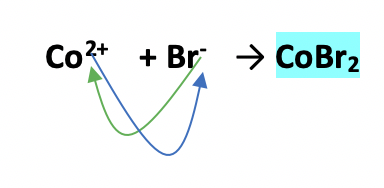# Problem: Determine the chemical formula of each of the following compounds and then use it to calculate the mass percent composition of each constituent element.cobalt(II) bromide

###### FREE Expert Solution

From the name cobalt (II) bromide,

• we have bromine as the anion with a charge of -1, Br-.
• we have cobalt, transition metal as the cation; based on the given name, it has a charge of +2, Co2+.

The chemical formula is:Step 1. Compute for the mass of each element for every 100 sample.

26.94 g Co

73.06 g Br

Step 2. Calculate percent composition.

84% (254 ratings)###### Problem Details

Determine the chemical formula of each of the following compounds and then use it to calculate the mass percent composition of each constituent element.

cobalt(II) bromide

Frequently Asked Questions

What scientific concept do you need to know in order to solve this problem?

Our tutors have indicated that to solve this problem you will need to apply the Mass Percent concept. You can view video lessons to learn Mass Percent. Or if you need more Mass Percent practice, you can also practice Mass Percent practice problems.